# Understanding LAG FunctionLAG

Definition: Returns the value from the past or from the future based on the syntax

Constraint: TIME & DATE Function. LAG will work only with Native TIME.

Syntax: LAG(X,Y,Z,O)

 Arguments Description Long Description Nature Format X Value to Offset Value to replace with a value froma different time period. Mandatory Any (Number, Boolean, Text, List, Time Period, Dates) Y Offset Amount Number of periods past, from which to retrieve a value. Positive values refer to past periods, negative to future ones and Zero is the current period. Mandatory Number Z Substitute Value Value to apply if the Offset amount specifies a period outside of the model's time range.Also used for non-positive offsets if you use the SEMISTRICT or STRICT keywords for the Non-positive behavior argument. Mandatory Any, based on X argument's format O Non Positive Behavior Determines if the LAG function should use Substitute Value if the Offset Amount is negative or zero Optional STRICT, SEMISTRICT, NONSTRICT

If you don't provide the fourth argument of syntax then the default behavior would be NONSTRICT.

STRICT: Returns the Value (X) if the Offset Value (Y) is positive. If (Y) is negative then it returns the Substitute Value(Z)

SEMISTRICT: Returns the Value (X) if the Offset Value (Y) is positive or zero. If (Y) is negative then it returns the Substitute Value(Z)

NONSTRICT: Returns the Value (X) in all cases. You don't need to provide this in an argument because it is considered to be Default behavior of Anaplan.

Now let's try to understand how the outputs change based on the arguments used

Scenario I: Hardcoding the Offset Amount.

In this example since the hardcoded value is +2 it pushes the Value two periods towards right or in another words it brings data from 2 periods in the past to the current period. Q1FY20 and Q2FY20 should have received inputs from Q3FY19 and Q4FY19 respectively but since the timescale starts from Q1FY20 here comes the concept of Substitute Value which is 0 in this case and that is the reason Q1 & Q2 have 0 as an output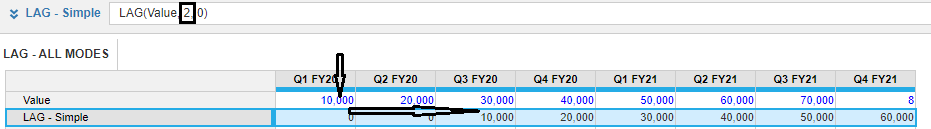Scenario II: Variable Offset & Substitute value using STRICT Mode.

In this example we are using STRICT Mode which means that if the Offset value is negative or zero then the Substitute value will be displayed otherwise it will bring the Value based on the Offset Amount. Red ones are where Substitute Values are being displayed  & the Green ones are where Values based on the Offset Amount are being shown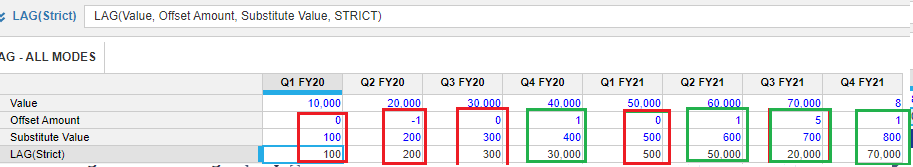Scenario III: Variable Offset & Substitute value using SEMISTRICT Mode.

In this example we are using SEMISTRICT Mode which means that if the Offset value is negative then the Substitute value will be displayed otherwise it will bring the Value based on the Offset Amount.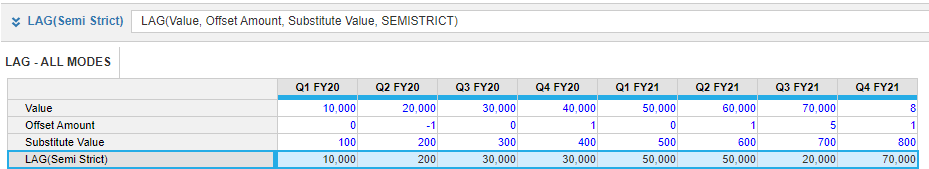Scenario IV: Variable Offset & Substitute value using NONSTRICT Mode.

In this example we are using NONSTRICT Mode which means Substitute value will not be displayed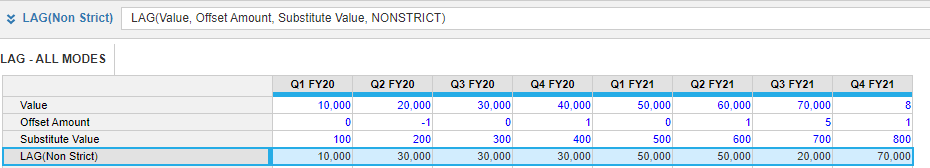Below grid for comparison of three modes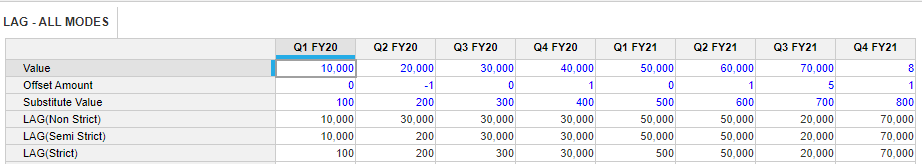Tagged: